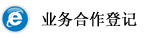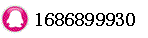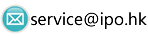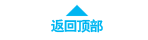# Discount Margin - DM时间：2018-05-02 19:15:38     浏览：56    评论：0

```
The return earned in addition to the index underlying the floating rate security.
|||The size of the discount margin depends on the price of the floating rate security. There are three basic situations:

1. If the price of a floater is equal to par, the investor's discount margin would be equal to the reset margin.

2. Due to the tendency for bond prices to converge to par as the bond reaches maturity, the investor can make an additional return over the reset margin if the floating rate bond was priced at a discount. The additional return plus the reset margin equals the discount margin.

3. Should the floating rate bond be priced above par, the discount margin would equal the reference rate less the reduced earnings.

```

0相关评论联系我们